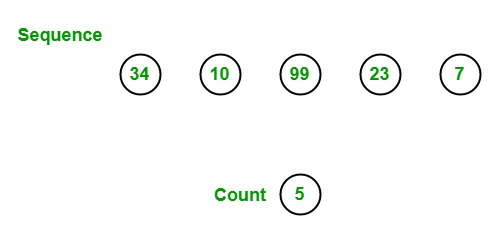# Count the number of element present in the sequence in LINQ?

• Last Updated : 21 May, 2019

In LINQ, you can count the total number of elements present in the given sequence by using the Count Method. This method returns the total number of elements present in the given sequence.This method can be overloaded in two different ways:

1. Count<TSource>(): This method returns the total number of elements present in the given specified sequence. The return type of this method is System.Int32. This method gives ArgumentNullException if the source is null and OverflowException if the value of the source is large. It does not support query syntax in C#, but you can wrap the query into brackets() and use aggregation functions as shown in Example 1. It supports query syntax in VB.NET.

Syntax:

`int Count<TSource>();`
2. Count<TSource>(Func<TSource, bool> predicate): This method is used to return the number of items which satisfy the given condition. The return type of this method is System.Int32. This method gives ArgumentNullException if the source or predicate is null and OverflowException if the value of the source is large.

Syntax:

`int Count<TSource>(Func<TSource, bool> predicate);`

Note: This method with predicate parameter is not supported in VB.NET.

Example 1:

 `// C# program to find total number of``// elements present in the given array``using` `System;``using` `System.Linq;`` ` `class` `GFG {`` ` `    ``// Main Method``    ``static` `public` `void` `Main()``    ``{`` ` `        ``// Data source``        ``int``[] sequence = {6, 455, 50, 56, 102,``                          ``89, 9, 100, 67, 29};`` ` `        ``// Display the sequence``        ``Console.WriteLine(``"The sequence is: "``);`` ` `        ``foreach``(``int` `s ``in` `sequence)``        ``{``            ``Console.WriteLine(s);``        ``}`` ` `        ``// Finding the total number of elements``        ``// present in the given sequence``        ``// Using Count function``        ``int` `result = sequence.Count();``        ``Console.WriteLine(``"Total number of Elements: {0}"``, result);``    ``}``}`
Output:
```The sequence is:
6
455
50
56
102
89
9
100
67
29
Total number of Elements: 10
```

Example 2:

 `// C# program to count the total``// number of the employees``using` `System;``using` `System.Linq;``using` `System.Collections.Generic;`` ` `// Employee details``public` `class` `Employee {`` ` `    ``public` `int` `emp_id``    ``{``        ``get``;``        ``set``;``    ``}`` ` `    ``public` `string` `emp_name``    ``{``        ``get``;``        ``set``;``    ``}`` ` `    ``public` `string` `emp_gender``    ``{``        ``get``;``        ``set``;``    ``}`` ` `    ``public` `string` `emp_hire_date``    ``{``        ``get``;``        ``set``;``    ``}`` ` `    ``public` `int` `emp_salary``    ``{``        ``get``;``        ``set``;``    ``}``}`` ` `class` `GFG {`` ` `    ``// Main method``    ``static` `public` `void` `Main()``    ``{``        ``List emp = ``new` `List() {``            ``new` `Employee() { emp_id = 209, emp_name = ``"Anjita"``, emp_gender = ``"Female"``, emp_hire_date = ``"12/3/2017"``, emp_salary = 20000 },``                ``new` `Employee() { emp_id = 210, emp_name = ``"Soniya"``, emp_gender = ``"Female"``, emp_hire_date = ``"22/4/2018"``, emp_salary = 30000 },``                ``new` `Employee() { emp_id = 211, emp_name = ``"Rohit"``, emp_gender = ``"Male"``, emp_hire_date = ``"3/5/2016"``, emp_salary = 40000 },``                ``new` `Employee() { emp_id = 212, emp_name = ``"Supriya"``, emp_gender = ``"Female"``, emp_hire_date = ``"4/8/2017"``, emp_salary = 40000 },``                ``new` `Employee() { emp_id = 213, emp_name = ``"Anil"``, emp_gender = ``"Male"``, emp_hire_date = ``"12/1/2016"``, emp_salary = 40000 },``                ``new` `Employee() { emp_id = 214, emp_name = ``"Anju"``, emp_gender = ``"Female"``, emp_hire_date = ``"17/6/2015"``, emp_salary = 50000 },``        ``};`` ` `        ``// Count the total number of employees``        ``// Using Count () method``        ``var` `res = (``from` `e ``in` `emp``                       ``select` `e.emp_id)``                      ``.Count();`` ` `        ``Console.WriteLine(``"Total number of Employees: {0}"``, res);``    ``}``}`
Output:
```Total number of Employees: 6
```

My Personal Notes arrow_drop_up